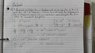# Finding the time at which the displacement is a Maximum - Calculus

• K.QMUL
In summary, the oscillator has a maxima and a minima at t=infinity, and the points of inflection are at t=0 and t=100 respectively.f

## Homework Statement

A damped oscillator has a displacement x as a function of time t given by x = e-t cos(∏t). Find the time at which the displacement is a maximum, when it is a minimum, and also nd
the time when x is a point of inflection.

x = e-t cos(∏t)

## The Attempt at a Solution

I've no problem differentiating the equation, its when I come to finding the value of T that I am having trouble with.

#### Attachments

•20131014_154740.jpg
36.5 KB · Views: 1,016
Well, you have identified that at t=infinity, there exists a stationary point. What is the displacement then?
A maximum or minimum?

You also have infinitely many other possibilities for stationary points, namely:
(pi)*sin(pi*t)+cos(pi*t)=0, meaning
tan(pi*t)=-1/pi

Which of these stationary points will represent maxima or minima?

When we use t=infinity the displacement (when substituting t=infinity in the equation) we get 0. The nature of the curve at this value is a 'Point of inflection' as shown in the attachment. Regarding the finding T for [∏sin(∏t) + cos(∏t) = 0] Arildno you put a minus sign in front of the ∏sin(∏t), shouldn't it be positive when looking through my workings out? When we do this we get Tan(∏t) = -1/∏.

Please do point out anything that seems incorrect so far...
-Thanks

#### Attachments

I hastily removed my minus sign, because it was, as you point out, incorrect.

## Homework Statement

A damped oscillator has a displacement x as a function of time t given by x = e-t cos(∏t). Find the time at which the displacement is a maximum, when it is a minimum, and also nd
the time when x is a point of inflection.

x = e-t cos(∏t)

## The Attempt at a Solution

I've no problem differentiating the equation, its when I come to finding the value of T that I am having trouble with.